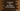# JavaScript program to find the largest of five numbers## Find the largest of five numbers in JavaScript :

In this tutorial, I will show you four different ways to find out the largest of five numbers using JavaScript. You can use these approaches to find out the largest of any n numbers. Let’s move to the examples :

## Example 1: Using if:

This is the simplest way to solve this problem. Use one if condition and compare each number with the others.

But the problem is that if you have n numbers, you will have to write n number of if statements. You can use this method for a small set of numbers, but for a large set of numbers, it is not a preferred way to solve.

Let’s move to the example program :

``````function getLargest(a, b, c, d, e) {
if (a > b && a > c && a > d && a > e) {
return a;
}
if (b > a && b > c && b > d && b > e) {
return b;
}
if (c > a && c > b && c > d && a > e) {
return c;
}
if (d > a && d > b && d > c && d > e) {
return d;
}
if (e > a && e > b && e > c && e > d) {
return e;
}
}

console.log(getLargest(1, 2, 3, 4, 5));
console.log(getLargest(2, 3, 4, 5, 1));
console.log(getLargest(3, 4, 5, 1, 2));
console.log(getLargest(4, 5, 1, 2, 3));
console.log(getLargest(5, 1, 2, 3, 4));``````

### Explanation :

• Here, getLargest JavaScript function is used to calculate the largest of five numbers i.e. the numbers we are taking as parameters.
• Inside this function, we have five if conditions. Each condition compares each of these numbers with the other numbers and returns that number if it is largest of them all.
• If the first number is the largest, then we will find the result in the first if block. If the last number is the largest, we will have to execute all if blocks to find out the result. That will be the worst case.

We are testing this function with five different sets of numbers. For each set, 5 is the largest number but it is placed in different positions.

### Output :

It will print the below output :

``````5;
5;
5;
5;
5;``````

## Example 2: Using a loop :

Another way to solve this problem in JavaScript is by using a loop. But, we need something to iterate if we use one loop. So, the idea is to put all of them in an array and iterate over that array.

``````function getLargest(a, b, c, d, e) {
let numArray = [b, c, d, e];
let largest = a;

for (let item of numArray) {
if (item > largest) {
largest = item;
}
}
return largest;
}

console.log(getLargest(1, 2, 3, 4, 5));
console.log(getLargest(2, 3, 4, 5, 1));
console.log(getLargest(3, 4, 5, 1, 2));
console.log(getLargest(4, 5, 1, 2, 3));
console.log(getLargest(5, 1, 2, 3, 4));``````

### Explanation :

We are using the same method name as the previous example.

• First of all, we are putting the numbers in an array numArray starting from the second number. Then, we are initializing one variable largest with the first number as its value. This variable will hold the largest number in the array.
• One for-of loop is used to iterate through the array values. On each iteration, we are checking if the current value is larger than largest or not, and accordingly, we are updating its value. In the end, variable largest will hold the largest among them.
• This function returns the variable largest. Note that you can also use any other looping methods.

### Output :

The output will be the same as the previous example.

## Example 3: Using Math.max() :

max() function of Math can take one array and return the largest of all :

``````function getLargest(a, b, c, d, e) {
let numArray = [a, b, c, d, e];

return Math.max(...numArray);
}

console.log(getLargest(1, 2, 3, 4, 5));
console.log(getLargest(2, 3, 4, 5, 1));
console.log(getLargest(3, 4, 5, 1, 2));
console.log(getLargest(4, 5, 1, 2, 3));
console.log(getLargest(5, 1, 2, 3, 4));``````

### Output :

As we are using the same input values, it will also print the same output.

## Example 4: Using sort :

We can sort an array using the inbuilt sort function. It takes one compare function and sorts the array based on it.

It sorts the array in place. In our case, we can sort the array in ascending order and return the last number using pop(). Ascending order sort will put the largest number at the end.

``````function getLargest(a, b, c, d, e) {
let numArray = [a, b, c, d, e];
return numArray.sort((a, b) => a - b).pop();
}

console.log(getLargest(1, 2, 3, 4, 5));
console.log(getLargest(2, 3, 4, 5, 1));
console.log(getLargest(3, 4, 5, 1, 2));
console.log(getLargest(4, 5, 1, 2, 3));
console.log(getLargest(5, 1, 2, 3, 4));``````

### Output :

``````5;
5;
5;
5;
5;``````

## Using reduce :

reduce() calculates one single value from an array. It takes one function as its parameter and calculates the final value using that function.

``````function getLargest(a, b, c, d, e) {
let numArray = [a, b, c, d, e];
return numArray.reduce((a, b) => (a > b ? a : b));
}

console.log(getLargest(1, 2, 3, 4, 5));
console.log(getLargest(2, 3, 4, 5, 1));
console.log(getLargest(3, 4, 5, 1, 2));
console.log(getLargest(4, 5, 1, 2, 3));
console.log(getLargest(5, 1, 2, 3, 4));``````

Here, a is the accumulator or the final value it is producing and b is the current value. We are checking if the current value is larger than the accumulator or not. If it is, we are setting the current value as accumulator. Finally, the accumulator holds the largest number and we are returning it.

### Output :

``````5;
5;
5;
5;
5;``````